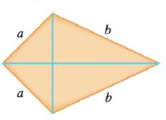Chapter 4.7, Problem 68E### Single Variable Calculus: Early Tr...

8th Edition
James Stewart
ISBN: 9781305270343

#### Solutions

Chapter
Section### Single Variable Calculus: Early Tr...

8th Edition
James Stewart
ISBN: 9781305270343
Textbook Problem

# The frame for a kite is to be made from six pieces of wood. The four exterior pieces have been cut with the lengths indicated in the figure. To maximize the area of the kite, how long should the diagonal pieces be?To determine

To find: The length of the diagonal pieces.

Explanation

Given:

Number of woods for the frame of the kites is 6.

Formula used:

Pythagorean Theorem:

If c denotes the length of the hypotenuse and a and b denote the length of the other two sides of a right angle triangle, then the Pythagorean theorem can be expressed as the Pythagorean equation: a2+b2=c2

Calculation

Consider the figure

In Figure 1,

In triangle ABC, ACD

By Pythagoras theorem,

BC=a2x2=CD

In triangle BCE,CDE

By Pythagoras theorem,

CE=a2x2x2+b2a2

Area of triangle ABC=12 (base)(height)

The area is

A= Area of triangle ABC + area of triangle ACD+ area of triangle BCE + area of triangle CDE

A=12(2a2x2)x+12(2a2x2)x2+b2a2=a2x2(x+x2+b2a2)

Differentiate with respect to x ,

For critical points,

Simplify that,

x(x2+b2a2)=a2x2x2(x2+b2a2)=a4+x42a2x2x4+b2x2a2x2=a4+x42a2x2b2x2+a2x2a4=0

Then¸

x2(a2+b2)=a4x=a2a2+b2

Differentiate dAdx with respect to x

### Still sussing out bartleby?

Check out a sample textbook solution.

See a sample solution

#### The Solution to Your Study Problems

Bartleby provides explanations to thousands of textbook problems written by our experts, many with advanced degrees!

Get Started

#### In Exercises 2934, rationalize the denominator of each expression. 33. a+bab

Applied Calculus for the Managerial, Life, and Social Sciences: A Brief Approach

#### For , f(x) = 0 2 3 f(3) does not exist

Study Guide for Stewart's Single Variable Calculus: Early Transcendentals, 8th

#### j × (−k) = i −i j + k −j − k

Study Guide for Stewart's Multivariable Calculus, 8th